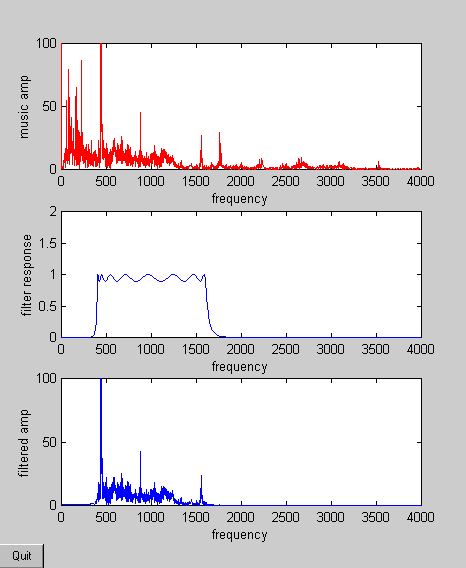Cornell University
ECE 5030
Using the Matlab DAQ toolbox

Introduction

The Data Aqusition (daq) Toolbox is a set of Matlab extensions which allow almost real-time control of external instruments and recording of electical signals. This toolbox has to handle the interface between a purely software environment (Matlab) and the real world of wires and voltages. There are several pieces to the interface:

• There has to be a chunk of hardware which attaches the real world to the computer. For the daq toolbox, this hardware can be either the Windows sound device or a variety of National Instruments (NI) data aqusition cards. The Windows sound device is limited, but can be used to input and output voltages in the frequency range of 50 to 5000 Hz and voltage range of about -1 to +1 volts. The NI cards are much more flexible.
• There is a piece of software which adapts the specific hardware to Matlab. This is referred to as a driver.
• There are the daq toolbox functions which allow a Matlab program to gain access to the hardware. This is the part you have to learn how to use.

The daq toolbox gives you a way to add computer control to your experiments. Possible uses for the daq toolbox include:

• An oscilloscope (running in a window on the PC) with specific analysis capabilities unavailable using a hardware scope, for example, optimum fitting of caridac potentials to a template.
• A stimulus generator with a waveform synthesized in Matlab, or modified from waveforms downloaded from the Web.
• An graphical user interface which allows you to control the parameters of an experiment.

Some more extensive virtual instruments are described on a separate web page.

Examples

1. Minimum necessary program (simpleAI.m) to record a voltage requires:
• Create an input object which couples Matlab to the hardware.
• Configure the input object by setting
• sample rate
• number of samples to obtain
• trigger method
• Start and trigger data collection
• Plot the input voltage (optional)
• Delete the object before exitting the program

2. Slow continuous input (SlowAnalogIn.m) of a voltage:
• Create an input object which couples Matlab to the hardware.
• grab one voltage data point
• Plot the input voltage (optional)
• Delete the object before exitting the program
3. Minimum necessary program (simpleAO.m) to produce a voltage output requires:
• Create an output object which couples Matlab to the hardware.
• Configure the output object by setting
• sample rate
• trigger method
• Start and trigger data output
• Plot the output voltage (optional)
• Delete the object before exitting the program

SimpleAO.m produces a computed series of waveforms. However, the program suffers from a short time gap (a few milliseconds) between each waveform. To obtain continuous tones, you can use the technique shown in simpleAOcontinuous.m. Here the waveforms are computed as separate columns of an array, then reshaped into one column for faster output. There is still a problem, however. There is a test near the end of the program which waits for output to finish before the output object is deleted. The code sits in this 'while loop' and does not let any other Matlab program execute. So if you are watching the output with a program like scope3 (described below) then scope3 freezes until simpleAOcontinuous.m is done. One solution is shown in simpleAOcontinuous2.m. Rather than using program code to wait for completion, the daq 'stopaction' is set to delete. This seems to work, although I can't find documentation to suggest that it should work. If you need to delete a bunch of analog outputs, but leave any existing inputs intact use:

```      existingAO=daqfind('name','winsound0-AO');
for i=1:length(existingAO)
delete(existingAO{i});
end
```
4. A simple oscilloscope using the Windows sound input (scope3.m). Note that this scope can set timebase and vertical gain, but the trigger is hardcoded. The coding is based on a GUI design scheme described in GUI Programming. The general scheme is to write a Matlab function which defines a bunch of GUI widgets in a window. The widgets (e.g. pushbutton) then recursively call the function when activated (e.g. pushed). Another version of the simple oscilloscope avoids the involuted style of scope3.m by writing the program as a loop which polls the various GUI controls. The major advantage is simplicity, the major disadvantage is loss of concurrency.

5. A program which reads the Windows sound card as fast as possible, filters the incoming signal, displays the filtered signal, and then echos the filtered signal out to the speakers. This program uses both analog input and analog output. To use it, you need to open up whatever utility controls the input and output to your sound card. On my Gateway there is an audio mixer control panel. The input (record input) should be set either to the CD player or to line-input (mini phonejack). The output (playback output) should be set to maximum amplitude on speakers and on wave output. If you use the CD player as an input, then you can have a analog input and output with no external wired connections. You can define a filter to be applied to the input, but since there is no user interface, you have to modify the code slightly to change the filter. The program opens one window with three subplots, shown below. The first subplot is the spectrum of the input signal. The second subplot shows the chosen filter frequency response. The third subplot is the spectrum of the input signal after it is filtered. The program is written as a simple loop which executes until a quit button in the lower left corner of the window is pressed. The loop repeats as soon as the analog output is complete.